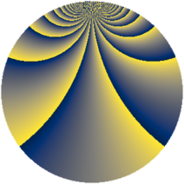# Properties

 Label 1002.2.eLevel $1002$ Weight $2$ Character orbit 1002.e Rep. character $\chi_{1002}(7,\cdot)$ Character field $\Q(\zeta_{83})$ Dimension $2296$ Sturm bound $336$

# Related objects

## Defining parameters

 Level: $$N$$ $$=$$ $$1002 = 2 \cdot 3 \cdot 167$$ Weight: $$k$$ $$=$$ $$2$$ Character orbit: $$[\chi]$$ $$=$$ 1002.e (of order $$83$$ and degree $$82$$) Character conductor: $$\operatorname{cond}(\chi)$$ $$=$$ $$167$$ Character field: $$\Q(\zeta_{83})$$ Sturm bound: $$336$$

## Dimensions

The following table gives the dimensions of various subspaces of $$M_{2}(1002, [\chi])$$.

Total New Old
Modular forms 14104 2296 11808
Cusp forms 13448 2296 11152
Eisenstein series 656 0 656

## Trace form

 $$2296q - 28q^{4} - 28q^{9} + O(q^{10})$$ $$2296q - 28q^{4} - 28q^{9} + 4q^{10} + 16q^{11} + 20q^{13} + 16q^{14} + 8q^{15} - 28q^{16} + 24q^{17} + 24q^{19} + 8q^{22} + 32q^{23} - 4q^{25} + 8q^{26} + 32q^{29} + 4q^{30} + 24q^{31} + 16q^{33} + 16q^{34} + 64q^{35} - 28q^{36} + 44q^{37} + 32q^{38} + 8q^{39} + 4q^{40} + 56q^{41} + 60q^{43} + 16q^{44} + 32q^{46} + 80q^{47} + 4q^{49} + 32q^{50} + 12q^{51} + 20q^{52} + 64q^{53} + 80q^{55} + 16q^{56} + 16q^{57} + 72q^{59} + 8q^{60} + 56q^{61} + 32q^{62} - 28q^{64} + 96q^{65} + 8q^{66} + 84q^{67} + 24q^{68} + 16q^{69} + 40q^{70} + 96q^{71} + 48q^{73} + 40q^{74} + 16q^{75} + 24q^{76} + 128q^{77} + 20q^{78} + 96q^{79} - 28q^{81} + 48q^{82} + 104q^{83} + 120q^{85} + 40q^{86} + 48q^{87} + 8q^{88} + 112q^{89} + 4q^{90} + 88q^{91} + 32q^{92} + 48q^{94} + 144q^{95} + 104q^{97} + 48q^{98} + 16q^{99} + O(q^{100})$$

## Decomposition of $$S_{2}^{\mathrm{new}}(1002, [\chi])$$ into newform subspaces

The newforms in this space have not yet been added to the LMFDB.

## Decomposition of $$S_{2}^{\mathrm{old}}(1002, [\chi])$$ into lower level spaces

$$S_{2}^{\mathrm{old}}(1002, [\chi]) \cong$$ $$S_{2}^{\mathrm{new}}(167, [\chi])$$$$^{\oplus 4}$$$$\oplus$$$$S_{2}^{\mathrm{new}}(334, [\chi])$$$$^{\oplus 2}$$$$\oplus$$$$S_{2}^{\mathrm{new}}(501, [\chi])$$$$^{\oplus 2}$$